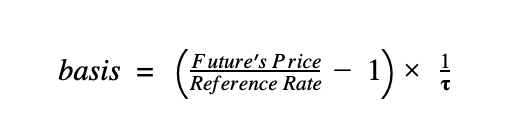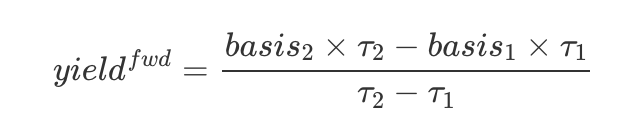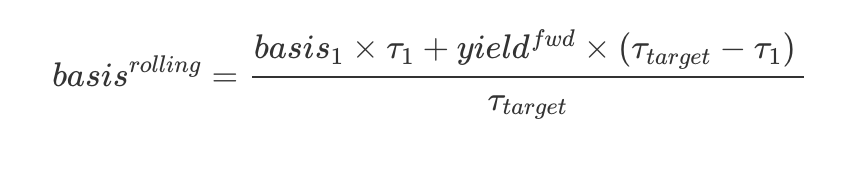# Basis

The basis is the annualized percent difference between the price of a theoretical futures contract and the price of its underlying spot market. Coin Metrics calculates this for several exchange-assets such as`binance-btc` and `ftx-eth`. We calculate four basis metrics at defined days to expiration.
Definition
The annualized basis metrics measure the difference between the spot price and a futures price for a contract with given time to expiration. This difference is calculated as a percentage of the underlying spot price which we use our reference rates to define, and annualized by the time to expiration.Methodology
We offer this calculation for various exchanges and assets in 30, 60, 90, and 120 day durations. The available contracts for an exchange and asset rarely ever align with the duration necessary and instead the target duration is calculated with a time adjusted weighting between the two contracts surrounding the target expiration. The target expiration is the time of the datapoint, t0, plus the indicated duration. For example if today was 2021-09-15 and we were creating a 30 day calculation then the target expiration would be 2021-10-15. In the case that the only futures contracts available for the asset and exchange combination were those with expirations on the last Friday of the month, we would use the contracts expiring on 2021-09-24 and 2021-10-29 as our two reference points. Please note that we currently utilized only quarterly contracts with expirations in March.
After defining the two contracts straddling the target expiration date, we find the basis between each contract and the spot price. Using the two contract’s basis we calculate the forward yield between the further dated contract and the nearer dated. This is the difference between the basis of each contract multiplied by their respective times to expiration, divided by the difference in their time to expiration.Using this forward yield, we then calculate the target date’s basis by multiplying the front contract’s basis by the time to expiration and adding to it the forward yield multiplied by the difference between the front contract and the target expiration. We divide this by the total target duration to arrive at the basis for our target duration.We currently calculate these metrics for the following exchange-asset combinations:
okex-btc, okex-eth, kraken-eth, huobi-eth, kraken-btc, huobi-btc, ftx-eth, deribit-eth, ftx-btc, deribit-btc, cme-btc, cme-eth, bitmex-btc, binance-btc, binance-eth